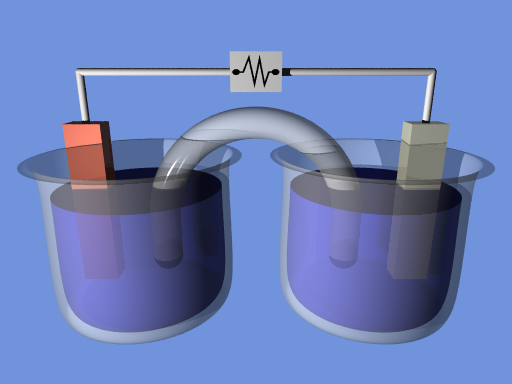## Free Energy and Cell Potential

#### Learning Objective

• Calculate the change in Gibbs free energy of an electrochemical cell, and discuss its implications for whether a redox reaction will be spontaneous

#### Key Points

• In a galvanic cell, the Gibbs free energy is related to the potential by: ΔG°cell = −nFE°cell.
• If E°cell > 0, then the process is spontaneous (galvanic cell).
• If E°cell < 0, then the process is nonspontaneous (electrolytic cell).

#### Terms

• electrode potentialThe potential difference of a half-reaction that occurs across a reversible cell made up of any electrode and a standard hydrogen electrode.
• galvanic cellElectrochemical cell that derives electrical energy from spontaneous redox reaction taking place within the cell.
• oxidizing agentAny substance that oxidizes, or receives electrons from, another; in so doing, it becomes reduced.
• Gibbs free energyThe difference between the enthalpy of a system and the product of its entropy and absolute temperature; a measure of the useful work obtainable from a thermodynamic system at constant temperature and pressure.

The basis for an electrochemical cell, such as the galvanic cell, is always a redox reaction that can be broken down into two half-reactions: oxidation occurs at the anode, where there is a loss of electrons, and reduction occurs at the cathode, where there is a gain of electrons. Electricity is generated due to the electric potential difference between two electrodes. This is related to how easily the oxidized species gives up electrons and how badly the reduced species wants to gain them.Electrochemical cellA demonstration electrochemical cell setup resembling the Daniell cell. The two half-cells are linked by a salt bridge carrying ions between them. Electrons flow in the external circuit.

In electrochemistry, the standard electrode potential, abbreviated E°, is the measure of the individual potential of a reversible electrode at standard state, which is with solutes at an effective concentration of 1 M, and gases at a pressure of 1 atm. The values are most often tabulated at 25 °C (298 K).

## Is It Oxidized or Reduced?

Since the standard electrode potentials are given in their ability to be reduced, the bigger the standard reduction potentials, the easier they are to be reduced; in other words, they are simply better oxidizing agents. For example, F2 has a potential of 2.87 V and Li+ has a potential of -3.05 V. F2 reduces easily and is therefore a good oxidizing agent. In contrast, Li(s) would rather undergo oxidation, so it is a good reducing agent.

In the example of Zn2+, whose standard reduction potential is -0.76 V, it can be oxidized by any other electrode whose standard reduction potential is greater than -0.76 V and can be reduced by any electrode with standard reduction potential less than -0.76 V.

## The Sign of the change in Gibbs Free Energy

In a galvanic cell, where a spontaneous redox reaction drives the cell to produce an electric potential, the change in Gibbs free energy must be negative. This is the opposite of the cell potential, which is positive when electrons flow spontaneously through the electrochemical cell. The relationship between $\Delta G^o$ and $E^o$ is given by the following equation:

$\Delta G^o = -nFE^o$

Here, n is the number of moles of electrons and F is the Faraday constant (96,485$\frac {Coulombs}{mole}$). As such, the following rules apply:

• If E°cell > 0, then the process is spontaneous (galvanic cell)
• If E°cell < 0, then the process is non-spontaneous (the voltage must be supplied, as in an electrolytic cell)

## Example

Calculate the change in Gibbs free energy of an electrochemical cell where the following redox reaction is taking place:

$Pb^{2+} + Ni \rightarrow Ni^{2+} + Pb \ \ \ \ \ \ \ \ E^o = 0.12\ V$

To solve, we need to use the equation:

$\Delta G^o = -nFE^o$

The number of moles of electrons transferred is 2, while the cell potential is equal to 0.12 V. One volt is $1\frac {Joule}{Coulomb}$.

$\Delta G^o = -2 \ moles\ e^- \times 96485\frac {Coulombs}{mole} \times 0.12 \frac {Joules}{Coulomb}$

$\Delta G^o = -23,156 \ J\ = -2.3 \ kJ$

Because the change in Gibbs free energy is negative, the redox process is spontaneous.